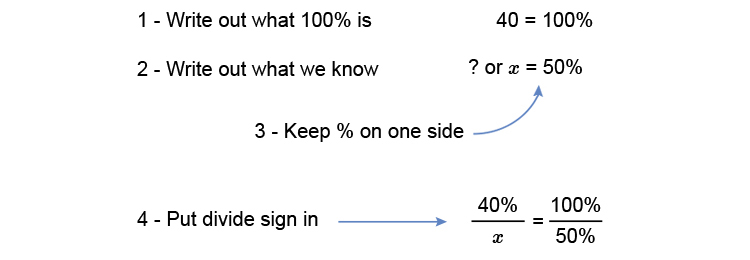# Percentage of a percentage

What is 50% of 40%?

You need to reword this slightly and say:

What is 50% of 40 ?

Now we can work this out.Multiply both sides by x to get x on its own.

(cancelxtimes40)/cancelx=(100timesx)/50

40=(100x)/50

40=2x

Divide both sides by 2 to get x on its own

40/2=(cancel2x)/cancel2

x=40/2

Answer: x=20%